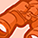# New to Qlik Sense

If you’re new to Qlik Sense, start with this Discussion Board and get up-to-speed quickly.

cancel
Showing results for
Did you mean:Contributor III

## Set analysis or IF not equal to any value from dimension

Hi,

I want to compare a column A with values, say value A to all possible values from column B, eventhough they are not related in the same row.

Column AColumn B
AX
BY
BZ
CA
CZ

For this example I want to compare COUNT(IF(Column B <> All possible values of Column A, 1)) This should result in 4.

I cannot find the syntax to compare to unrelated values.

1 Solution

Accepted SolutionsMVP

Or this

=Count([Column A]) - Count({<[Column A] = p([Column B])>} [Column A])

2 RepliesMVP

May be this

Count({<[Column A] = e([Column B])>} [Column A])MVP

Or this

=Count([Column A]) - Count({<[Column A] = p([Column B])>} [Column A])